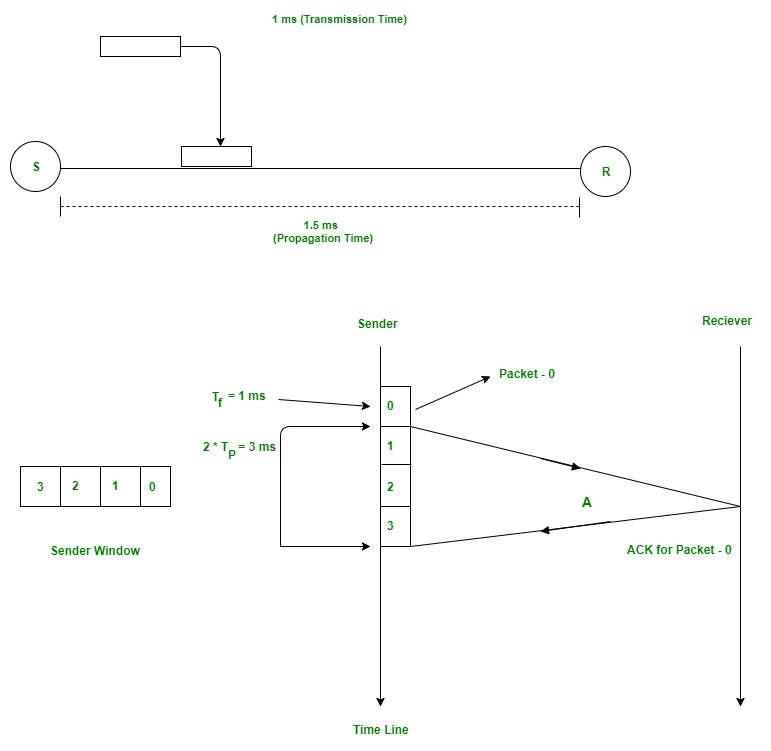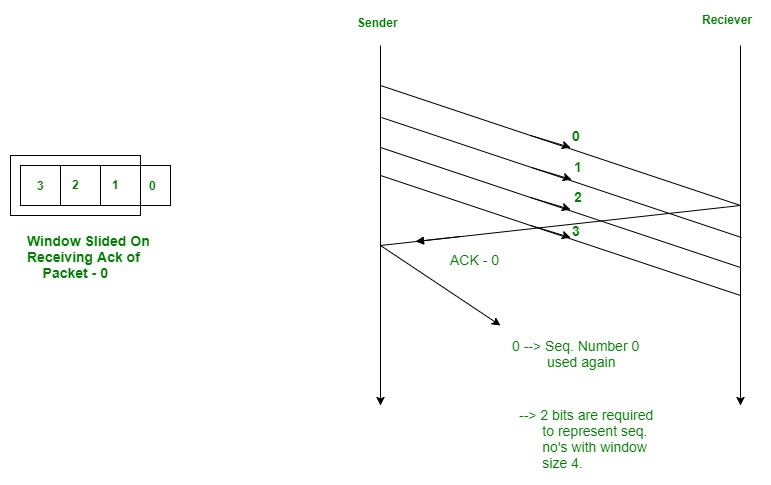Open in App
Not now

# Sliding Window Protocol | Set 1 (Sender Side)

• Difficulty Level : Medium
• Last Updated : 25 Mar, 2023

Prerequisite : Stop and Wait ARQ The Stop and Wait ARQ offers error and flow control, but may cause big performance issues as sender always waits for acknowledgement even if it has next packet ready to send. Consider a situation where you have a high bandwidth connection and propagation delay is also high (you are connected to some server in some other country through a high-speed connection), you can’t use this full speed due to limitations of stop and wait. Sliding Window protocol handles this efficiency issue by sending more than one packet at a time with a larger sequence number. The idea is same as pipelining in architecture.

## Few Terminologies :

Transmission Delay (Tt) – Time to transmit the packet from host to the outgoing link. If B is the Bandwidth of the link and D is the Data Size to transmit

`    Tt = D/B  `

Propagation Delay (Tp) – It is the time taken by the first bit transferred by the host onto the outgoing link to reach the destination. It depends on the distance d and the wave propagation speed s (depends on the characteristics of the medium).

`   Tp = d/s  `

Efficiency – It is defined as the ratio of total useful time to the total cycle time of a packet. For stop and wait protocol,

```Total cycle time = Tt(data) + Tp(data) +
Tt(acknowledgement) + Tp(acknowledgement)
=  Tt(data) + Tp(data) + Tp(acknowledgement)
=   Tt + 2*Tp```

Since acknowledgements are very less in size, their transmission delay can be neglected.

```Efficiency = Useful Time / Total Cycle Time
= Tt/(Tt + 2*Tp) (For Stop and Wait)
= 1/(1+2a)  [ Using a = Tp/Tt ]```

Effective Bandwidth(EB) or Throughput – Number of bits sent per second.

```EB = Data Size(D) / Total Cycle time(Tt + 2*Tp)
Multiplying and dividing by Bandwidth (B),
=  (1/(1+2a)) * B   [ Using a = Tp/Tt ]
=  Efficiency * Bandwidth```

Capacity of link – If a channel is Full Duplex, then bits can be transferred in both the directions and without any collisions. Number of bits a channel/Link can hold at maximum is its capacity.

``` Capacity = Bandwidth(B) * Propagation(Tp)

For Full Duplex channels,
Capacity = 2*Bandwidth(B) * Propagation(Tp)```

## Concept Of Pipelining

In Stop and Wait protocol, only 1 packet is transmitted onto the link and then sender waits for acknowledgement from the receiver. The problem in this setup is that efficiency is very less as we are not filling the channel with more packets after 1st packet has been put onto the link. Within the total cycle time of Tt + 2*Tp units, we will now calculate the maximum number of packets that sender can transmit on the link before getting an acknowledgement.

``` In Tt units ----> 1 packet is Transmitted.
In 1 units  ----> 1/Tt packet can be Transmitted.
In  Tt + 2*Tp units ----->  (Tt + 2*Tp)/Tt
packets can be Transmitted
------>  1 + 2a  [Using a = Tp/Tt]```

Maximum packets That can be Transmitted in total cycle time = 1+2*a Let me explain now with the help of an example. Consider Tt = 1ms, Tp = 1.5ms. In the picture given below, after sender has transmitted packet 0, it will immediately transmit packets 1, 2, 3. Acknowledgement for 0 will arrive after 2*1.5 = 3ms. In Stop and Wait, in time 1 + 2*1.5 = 4ms, we were transferring one packet only. Here we keep a window of packets that we have transmitted but not yet acknowledged.After we have received the Ack for packet 0, window slides and the next packet can be assigned sequence number 0. We reuse the sequence numbers which we have acknowledged so that header size can be kept minimum as shown in the diagram given below.## Minimum Number Of Bits For Sender window (Very Important For GATE)

As we have seen above,

``` Maximum window size = 1 + 2*a    where a = Tp/Tt

Minimum sequence numbers required = 1 + 2*a. ```

Efficiency: The sliding window protocol is an efficient method of transmitting data across a network because it allows multiple packets to be transmitted at the same time. This increases the overall throughput of the network.

Reliable: The protocol ensures reliable delivery of data, by requiring the receiver to acknowledge receipt of each packet before the next packet can be transmitted. This helps to avoid data loss or corruption during transmission.

Flexibility: The sliding window protocol is a flexible technique that can be used with different types of network protocols and topologies, including wireless networks, Ethernet, and IP networks.

Congestion Control: The sliding window protocol can also help control network congestion by adjusting the size of the window based on the network conditions, thereby preventing the network from becoming overwhelmed with too much traffic.